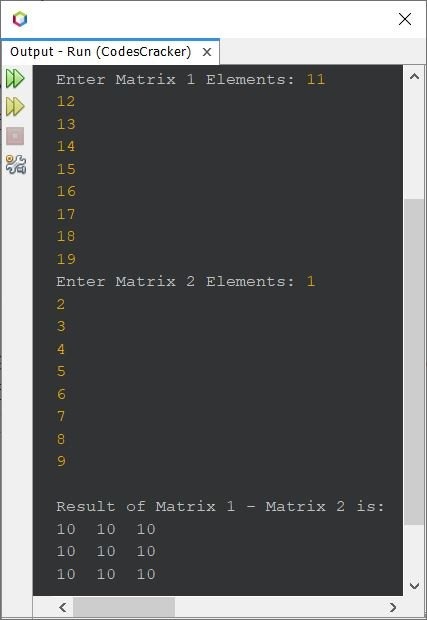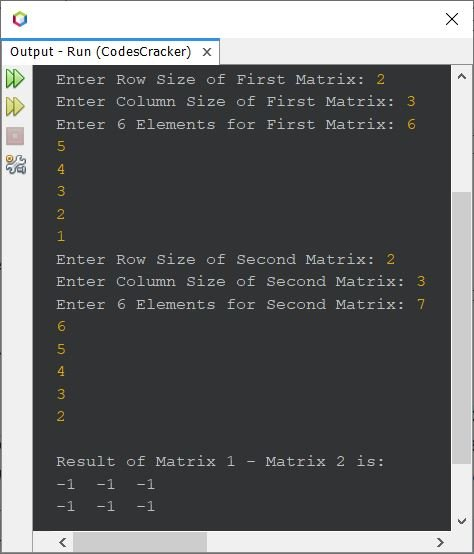# Java Program to Subtract Two Matrices

This article covers a program in Java that perform matrix subtraction. Two programs are included in this article, one to perform subtraction on 3*3 matrices, and other to perform subtraction based on given order and elements.

If you're not aware about, how the matrix subtraction performs ?
then refer to Matrix Subtraction. Now let's create the program.

## Subtract Two 3*3 Matrices in Java

The question is, write a Java program to subtract two 3*3 matrices. Elements of both matrices must be received by user at run-time of the program. The program given below is its answer:

```import java.util.Scanner;

public class CodesCracker
{
public static void main(String[] args)
{
int i, j;
int[][] mat1 = new int;
int[][] mat2 = new int;
int[][] mat3 = new int;
Scanner scan = new Scanner(System.in);

System.out.print("Enter Matrix 1 Elements: ");
for(i=0; i<3; i++)
{
for(j=0; j<3; j++)
{
mat1[i][j] = scan.nextInt();
}
}
System.out.print("Enter Matrix 2 Elements: ");
for(i=0; i<3; i++)
{
for(j=0; j<3; j++)
{
mat2[i][j] = scan.nextInt();
}
}

// subtracting matrices
for(i=0; i<3; i++)
{
for(j=0; j<3; j++)
{
mat3[i][j] = mat1[i][j] - mat2[i][j];
}
}

System.out.println("\nResult of Matrix 1 - Matrix 2 is:");
for(i=0; i<3; i++)
{
for(j=0; j<3; j++)
{
System.out.print(mat3[i][j]+ "  ");
}
System.out.print("\n");
}
}
}```

The snapshot given below shows the sample run of above program, with user input 11, 12, 13, 14, 15, 16, 17, 18, 19 as matrix 1 elements, and 1, 2, 3, 4, 5, 6, 7, 8, 9 as matrix 2 elements:That is,

```11  12  13       1  2  3       10  10  10
14  15  16   -   4  5  6   =   10  10  10
17  18  19       7  8  9       10  10  10```

## Subtract Two Matrices of Given Order in Java

Here is the modified version of previous program. This program allows user to define the order of both the matrices too, along with its elements, to subtract second matrix from first.

```import java.util.Scanner;

public class CodesCracker
{
public static void main(String[] args)
{
int i, j;
Scanner scan = new Scanner(System.in);

System.out.print("Enter Row Size of First Matrix: ");
int rOne = scan.nextInt();
System.out.print("Enter Column Size of First Matrix: ");
int cOne = scan.nextInt();
int[][] mat1 = new int[rOne][cOne];
System.out.print("Enter " +(rOne*cOne)+ " Elements for First Matrix: ");
for(i=0; i<rOne; i++)
{
for(j=0; j<cOne; j++)
mat1[i][j] = scan.nextInt();
}
System.out.print("Enter Row Size of Second Matrix: ");
int rTwo = scan.nextInt();
System.out.print("Enter Column Size of Second Matrix: ");
int cTwo = scan.nextInt();
int[][] mat2 = new int[rTwo][cTwo];
System.out.print("Enter " +(rTwo*cTwo)+ " Elements for Second Matrix: ");
for(i=0; i<rTwo; i++)
{
for(j=0; j<cTwo; j++)
mat2[i][j] = scan.nextInt();
}

if(rOne==rTwo && cOne==cTwo)
{
int[][] mat3 = new int[rOne][cOne];
for(i=0; i<rOne; i++)
{
for(j=0; j<cOne; j++)
mat3[i][j] = mat1[i][j] - mat2[i][j];
}

System.out.println("\nResult of Matrix 1 - Matrix 2 is:");
for(i=0; i<rOne; i++)
{
for(j=0; j<cOne; j++)
System.out.print(mat3[i][j]+ "  ");
System.out.print("\n");
}
}
else
System.out.println("\nOrder Mismatched!");
}
}```

The sample run of above program with user input 2 as row size and 3 as column size for both matrices, 6, 5, 4, 3, 2, 1 as six elements for first matrix, and 7, 6, 5, 4, 3, 2 as six elements for second matrix, is shown in the snapshot given below:#### Same Program in Other Languages

Java Online Test

« Previous Program Next Program »